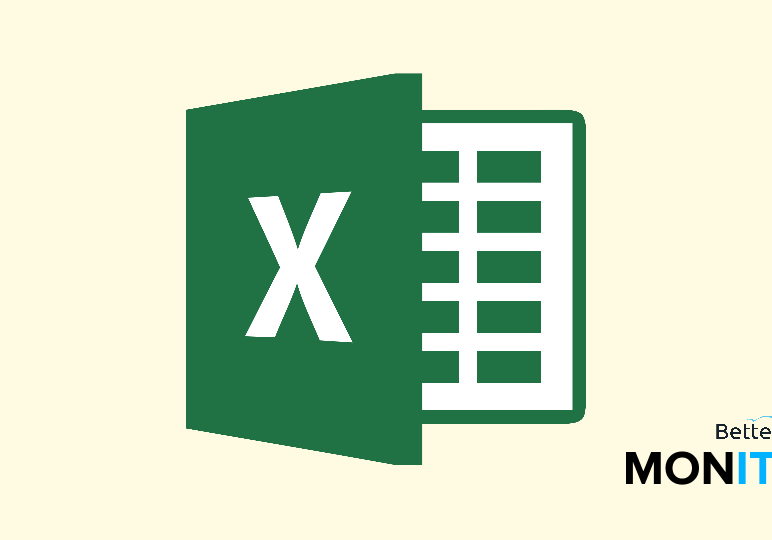## The Top 8 Excel Functions You Should Know## IF

This post is an excerpt from Popular Functions in Excel: Lesson 1 – “IF.”

“IF” checks whether a condition is met and then returns different values based on whether it’s TRUE or FALSE.

## SUM

This post is an excerpt from Popular Functions in Excel: Lesson 2 – “SUM.”

“SUM” adds values together and really becomes invaluable when working with large data sets.

## COUNTIF

This post is an excerpt from Popular Functions in Excel: Lesson 3 – “COUNTIF.”

The “COUNTIF” function counts the number of cells that meet a certain criteria. You can gather a tally of certain types of cells using a logical IF condition as part of the function.

## VLOOKUP

This post is an excerpt from Popular Functions in Excel: Lesson 4 – “VLOOKUP.”

Ah, VLOOKUP. This function, short for “vertical lookup,” is very useful for finding specific information in your worksheet.

## SUMIF

This post is an excerpt from Popular Functions in Excel: Lesson 5 – “SUMIF.”

The “SUMIF” function adds values in a range that meet a specified criteria. It offers the functionality of the SUM command but allows you to combine it with conditional terms–i.e., only IF your specific criteria is met will Excel go ahead and execute the sum.

## LOOKUP

This post is an excerpt from Popular Functions in Excel: Lesson 6 – “LOOKUP.”

“LOOKUP” is useful when you want to search only a single row or column, and find the information in a corresponding spot from somewhere else in your spreadsheet.

## CONCATENATE

This post is an excerpt from Popular Functions in Excel: Lesson 7 – “CONCATENATE.”

The “CONCATENATE” function combines text from different cells into one cell.

## MATCH

This post is an excerpt from Popular Functions in Excel: Lesson 8 – “MATCH.”

The “MATCH” function gives you the position of an item in a range.